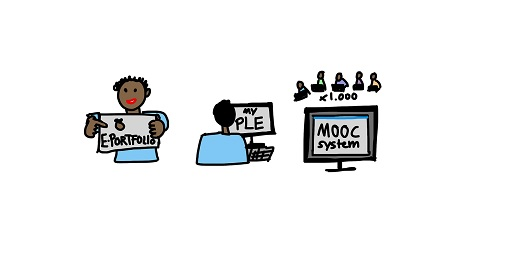# [analyticsvidhya] BigDataFr recommends: 19 MOOCs on Mathematics & Statistics for Data Science & Machine LearningBigDataFr recommends: 19 MOOCs on Mathematics & Statistics for Data Science & Machine Learning

[…] Introduction

Mathematics & Statistics are the founding steps for data science and machine learning. Most of the successful data scientists I know of, come from one of these areas – computer science, applied mathematics & statistics or economics. If you wish to excel in data science, you must have a good understanding of basic algebra and statistics.

However, learning Maths for people not having background in mathematics can be intimidating. First, you have to identify what to study and what not. The list can include Linear Algebra, calculus, probability, statistics, discrete mathematics, regression, optimization and many more topics. What do you do? How deep to you want to get in each of these topics? It is very difficult to navigate through this by yourself.

Get started now to learn & explore mathematics for data science. […]